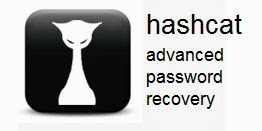Hashcat plus is Worlds first and only GPGPU based rule engine and Worlds fastest md5crypt, phpass, mscash2 and WPA / WPA2 cracker.
Features Free Multi-GPU (up to 16 gpus) Multi-Hash (up to 24 million hashes) Multi-OS (Linux & Windows native binaries) Multi-Platform (OpenCL & CUDA support) Multi-Algo (see below) Low resource utilization, you can still watch movies or play games while cracking Focuses highly iterated, modern hashes Focuses single dictionary based attacks Supports pause / resume while cracking Supports reading words from file Supports reading words from stdin Integrated thermal watchdog 20+ Algorithms implemented with performance in mind ... and much more

## Usage ::

```Usage: ./hashcat-cli64.bin [options] hashfile [wordfiles|directories]

Startup:
-V,  --version                     print version
-h,  --help                        print help
--eula                        print eula

Logging and Files:
--remove                      enable remove of hash from hashlist once it is cracked
--quiet                       suppress output
--stdout                      stdout mode
--disable-potfile             do not write potfile
-r,  --rules-file=FILE             rules-file for hybrid-attack
-o,  --output-file=FILE            output-file for recovered hashes
--output-format=NUM           0 = hash:pass
1 = hash:hex_pass
2 = hash:pass:hex_pass
-e,  --salt-file=FILE              salts-file for unsalted hashlists
--debug-file=FILE             debug-file
--debug-mode=NUM              1 = save finding rule (hybrid only)
2 = save original word (hybrid only)
-p,  --seperator-char=CHAR         seperator-char for hashlists

Resources:

-c,  --segment-size=NUM            number of mb to cache from wordfile
-s,  --words-skip=NUM              skip number of words (for resume)
-l,  --words-limit=NUM             limit number of words (for distributed)

Attacks:
-g,  --generate-rules=NUM          number of self-generating rules
--generate-rules-func-min=NUM force number of functions per rule min
--generate-rules-func-max=NUM force number of functions per rule max
-a,  --attack-mode=NUM             number of attack-mode
0 = Straight *
1 = Combination *
2 = Toggle-Case
3 = Brute-Force
4 = Permutation
5 = Table-Lookup
* = for Hybrid-Attack use -r or -g

-m,  --hash-mode=NUM               number of hash-mode

0 = MD5                           200 = MySQL
1 = md5(\$pass.\$salt)              300 = MySQL4.1/MySQL5
2 = md5(\$salt.\$pass)              400 = MD5(Wordpress)
3 = md5(md5(\$pass))               400 = MD5(phpBB3)
4 = md5(md5(md5(\$pass)))          500 = MD5(Unix)
5 = vBulletin < v3.8.5            600 = SHA-1(Base64)
6 = md5(md5(\$salt).\$pass)         700 = SSHA-1(Base64)
7 = md5(\$salt.md5(\$pass))         800 = SHA-1(Django)
8 = md5(\$salt.\$pass.\$salt)        900 = MD4
9 = md5(md5(\$salt).md5(\$pass))   1000 = NTLM
10 = md5(md5(\$pass).md5(\$salt))   1100 = Domain Cached Credentials
11 = md5(\$salt.md5(\$salt.\$pass))  1200 = MD5(Chap)
12 = md5(\$salt.md5(\$pass.\$salt))  1300 = MSSQL
15 = vBulletin > v3.8.5
31 = md5(strtoupper(md5(\$pass)))
100 = SHA1                         1400 = SHA256
101 = sha1(\$pass.\$salt)            1600 = MD5(APR)
102 = sha1(\$salt.\$pass)            1700 = SHA512
103 = sha1(sha1(\$pass))            1800 = SHA-512(Unix)
104 = sha1(sha1(sha1(\$pass)))

Toggle-Case specific:
--toggle-min=NUM               number of alphas in plain minimum
--toggle-max=NUM               number of alphas in plain maximum

Brute-Force specific:
--bf-cs-buf=CHARS              charset for attack

Permutation specific:
--perm-min=NUM                 number of chars in plain minimum
--perm-max=NUM                 number of chars in plain maximum

Table-Lookup specific:
--table-min=NUM                number of chars in plain minimum
--table-max=NUM                number of chars in plain maximum
--table-file=FILE              table file```
` `
` `
```
```
` `